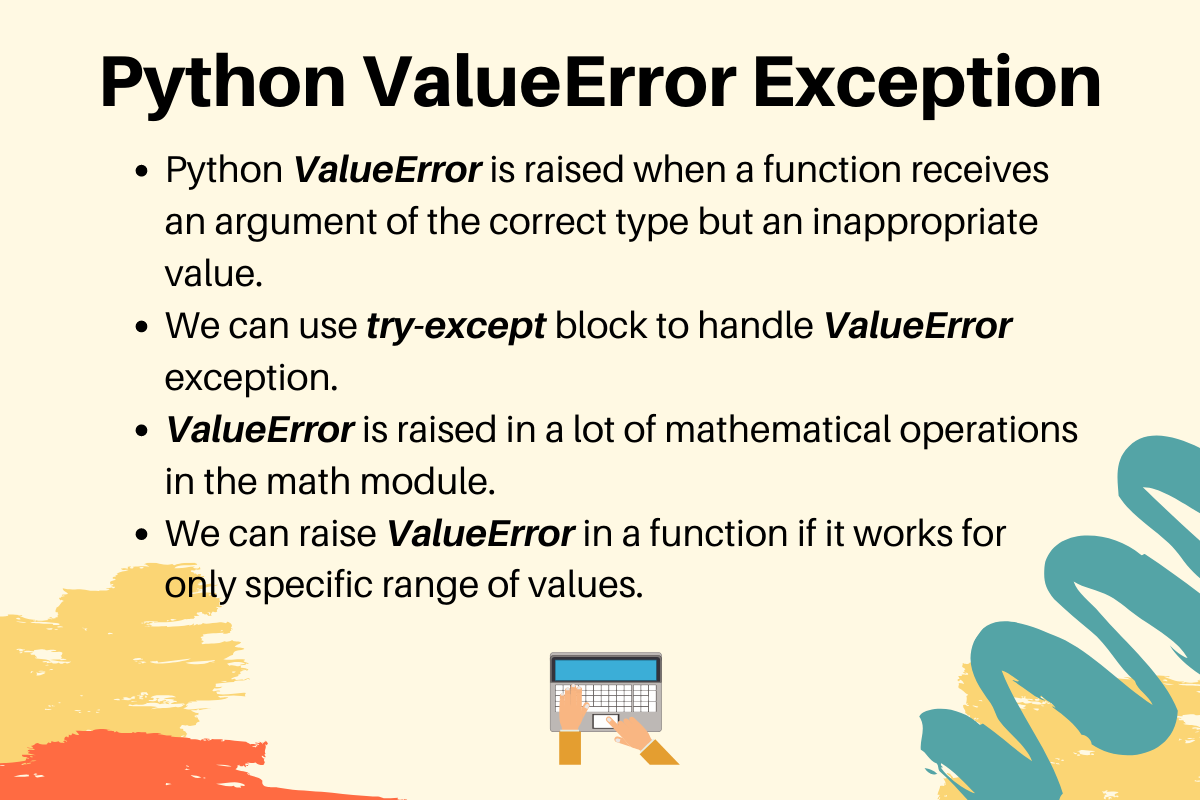# Python ValueError Exception Handling Examples

Published on August 3, 2022By PankajWhile we believe that this content benefits our community, we have not yet thoroughly reviewed it. If you have any suggestions for improvements, please let us know by clicking the “report an issue“ button at the bottom of the tutorial.

## 1. What is Python ValueError?

Python ValueError is raised when a function receives an argument of the correct type but an inappropriate value. Also, the situation should not be described by a more precise exception such as IndexError.

## 2. ValueError Example

You will get ValueError with mathematical operations, such as square root of a negative number.

``````>>> import math
>>>
>>> math.sqrt(-10)
Traceback (most recent call last):
File "<stdin>", line 1, in <module>
ValueError: math domain error
>>>
``````

## 3. Handling ValueError Exception

Here is a simple example to handle ValueError exception using try-except block.

``````import math

x = int(input('Please enter a positive number:\n'))

try:
print(f'Square Root of {x} is {math.sqrt(x)}')
except ValueError as ve:
print(f'You entered {x}, which is not a positive number.')
``````

Here is the output of the program with different types of input.

``````Please enter a positive number:
16
Square Root of 16 is 4.0

-10
You entered -10, which is not a positive number.

abc
Traceback (most recent call last):
File "/Users/pankaj/Documents/PycharmProjects/hello-world/journaldev/errors/valueerror_examples.py", line 11, in <module>
x = int(input('Please enter a positive number:\n'))
ValueError: invalid literal for int() with base 10: 'abc'
``````

Our program can raise ValueError in int() and math.sqrt() functions. So, we can create a nested try-except block to handle both of them. Here is the updated snippet to take care of all the ValueError scenarios.

``````import math

try:
x = int(input('Please enter a positive number:\n'))
try:
print(f'Square Root of {x} is {math.sqrt(x)}')
except ValueError as ve:
print(f'You entered {x}, which is not a positive number.')
except ValueError as ve:
print('You are supposed to enter positive number.')
``````

## 4. Raising ValueError in a function

Here is a simple example where we are raising ValueError for input argument of correct type but inappropriate value.

``````import math

def num_stats(x):
if x is not int:
raise TypeError('Work with Numbers Only')
if x < 0:
raise ValueError('Work with Positive Numbers Only')

print(f'{x} square is {x * x}')
print(f'{x} square root is {math.sqrt(x)}')
``````

## 5. References

Thanks for learning with the DigitalOcean Community. Check out our offerings for compute, storage, networking, and managed databases.Pankaj

author

#### Still looking for an answer?

Ask a questionSearch for more help

JournalDev
DigitalOcean EmployeeSeptember 25, 2020

Wow!! You codes are simple hence easy to understand… they are really fun to type…thanks

- Jemoh

Click below to sign up and get \$200 of credit to try our products over 60 days!

### Popular Topics##### Hollie's Hub for Good

Working on improving health and education, reducing inequality, and spurring economic growth? We’d like to help.##### Become a contributor

You get paid; we donate to tech nonprofits.

##### Welcome to the developer cloud

DigitalOcean makes it simple to launch in the cloud and scale up as you grow – whether you’re running one virtual machine or ten thousand.© 2023 DigitalOcean, LLC.